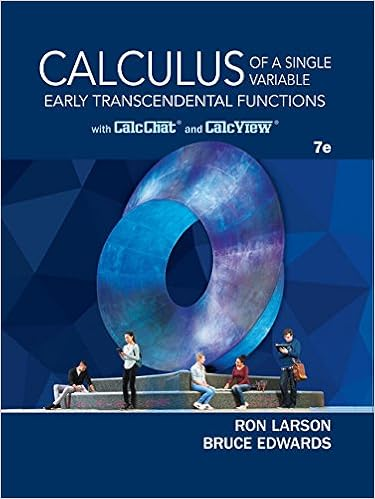# Now we compute the left side of equation 2 by

• Notes
• mimi304
• 86
• 100% (2) 2 out of 2 people found this document helpful

This preview shows page 31 - 33 out of 86 pages.

##### We have textbook solutions for you!
The document you are viewing contains questions related to this textbook.The document you are viewing contains questions related to this textbook.
Chapter 7 / Exercise 35
Calculus of a Single Variable: Early Transcendental Functions
Edwards/LarsonExpert Verified
Now we compute the left side of Equation 2 by breaking up as the union of the four curves , , , and shown in Figure 3. On we take as the parameter and write the parametric equations as , , . Thus Observe that goes from right to left but goes from left to right, so we can write the parametric equations of as , , . Therefore On or (either of which might reduce to just a single point), is constant, so and Hence D 2 y C P dx yy D P y dA 3 y C Q dy yy D Q x dA D D x , y a x b , t 1 x y t 2 x t 1 t 2 4 yy D P y dA y b a y t 2 x t 1 x P y x , y dy dx y b a P x , t 2 x P x , t 1 x dx C C 1 C 2 C 3 C 4 C 1 x x x y t 1 x a x b y C 1 P x , y dx y b a P x , t 1 x dx C 3 C 3 C 3 x x y t 2 x a x b y C 3 P x , y dx y C 3 P x , y dx y b a P x , t 2 x dx C 2 C 4 x dx 0 y C 2 P x , y dx 0 y C 4 P x , y dx y C P x , y dx y C 1 P x , y dx y C 2 P x , y dx y C 3 P x , y dx y C 4 P x , y dx y b a P x , t 1 x dx y b a P x , t 2 x dx George Green Green’s Theorem is named after the self- taught English scientist George Green (1793–1841). He worked full-time in his father’s bakery from the age of nine and taught himself mathematics from library books. In 1828 he published privately An Essay on the Application of Mathematical Analysis to the Theories of Electricity and Magnetism , but only 100 copies were printed and most of those went to his friends. This pamphlet contained a theorem that is equivalent to what we know as Green’s Theorem, but it didn’t become widely known at that time. Finally, at age 40, Green entered Cambridge University as an undergraduate but died four years after graduation. In 1846 William Thomson (Lord Kelvin) located a copy of Green’s essay, realized its significance, and had it reprinted. Green was the first person to try to formulate a mathematical theory of elec- tricity and magnetism. His work was the basis for the subsequent electromagnetic theories of Thomson, Stokes, Rayleigh, and Maxwell. FIGURE 3 y x 0 a b D y=g™(x) y=g¡(x) C™
##### We have textbook solutions for you!
The document you are viewing contains questions related to this textbook.The document you are viewing contains questions related to this textbook.
Chapter 7 / Exercise 35
Calculus of a Single Variable: Early Transcendental Functions
Edwards/LarsonExpert Verified
1110 CHAPTER 16 Comparing this expression with the one in Equation 4, we see that Equation 3 can be proved in much the same way by expressing as a type II region (see Exercise 30). Then, by adding Equations 2 and 3, we obtain Green’s Theorem. Evaluate , where is the triangular curve consisting of the line segments from to , from to , and from to . SOLUTION Although the given line integral could be evaluated as usual by the methods of Section 16.2, that would involve setting up three separate integrals along the three sides of the triangle, so let’s use Green’s Theorem instead. Notice that the region enclosed by is simple and has positive orientation (see Figure 4). If we let and , then we have Evaluate , where is the circle . SOLUTION The region bounded by is the disk , so let’s change to polar coordinates after applying Green’s Theorem: In Examples 1 and 2 we found that the double integral was easier to evaluate than the line integral. (Try setting up the line integral in Example 2 and you’ll soon be convinced!) But sometimes it’s easier to evaluate the line integral, and Green’s Theorem is used in the reverse direction. For instance, if it is known that
•••